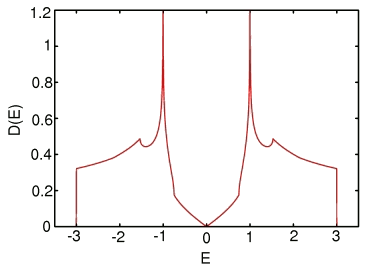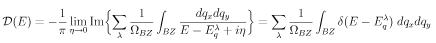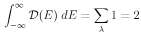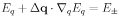Graphene Density of States

R. B. Laughlin May 19, 2008

(Submitted as coursework for Physics 373, Stanford University, Spring 2008)Fig. 1: Density of states for idealized graphene. The computer code that generated this is available here. I plotted using gnuplot. The plot file is shown here. The units are states per unit energy per unit cell. The function is properly normalized to 2.

This is a report template that shows how the band structure of graphene calculated in my previous report is converted to a density of states, perwhere λ sums over the two bands (upper and lower) and ΩBZ denotes the brillouin zone volume. This normalization gives states per unit cell, and thus in the case of graphene gives ussince there are two bands. Evaluating a density of states quickly and with sharp convergence is central to evaluating Feynman graphs numerically. As one can see in Fig. 1, it has slope discontinuities and singularities reminiscent of the logarithmic branch cuts you see in the Lindhard function. This is not an accident, as they're physically the same thing. In the band structure literature, these features are called van hove singularities. It is essential to get them accurately right with minimal computer time. The calculation that generated this figure took less than 1 second to run.

Although you can, in principle, just sum resonant denominators, it doesn't work in practice because the convergence is poor. You need too many momentum-space points. The attached code explains succinctly how the calculation work. Here, however is the basic idea:Create a fine accumulation array for the function. In this case I chose 1000 energy bins between 0 and 3.5. Zero out this array.Loop over a regularly spaced grid in the brillouin zone. For this momentum, calculate both the energy of the band and the gradient of this energy with respect to q. The latter is usually easy to do analytically. This gradient is a Taylor expansion of the band valid in the immediate vicinty of the point in questionFor each momentum grid point, loop over the energy bins. Each bin spans a narrow energy range, let's say between E+ and E-. Each of these generates a line in momentum space, perThese two lines bound a ribbon in momentum space. Now calculate the intersection of this ribbon with the little box surrounding your momentum point. The result is a small area in momentum space. Note that most of the time this area is zero because ribbon doesn't intersect your little box.Now add this area to whatever is in the energy bin accumulator already. The function you are accumulating has units of momentum space volume.When you are done with all these loops, go back and divide the contents of each bin by the brillouin zone area and the energy bin spacing.

The LaTeX source for the above equations is available here.